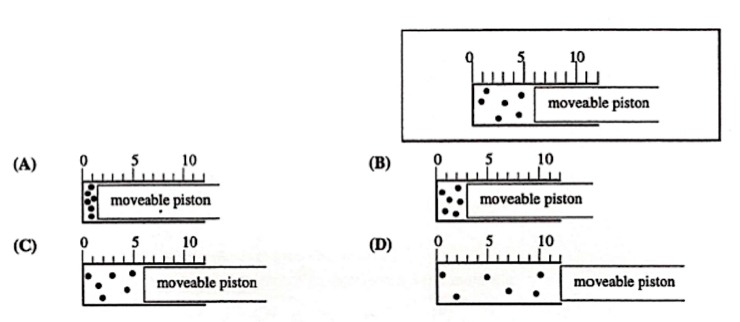# Problem: Which diagram best represents the final system, if the pressure of the gas in this cylinder were doubled and the temperature increased from 200 K to 400 K?

99% (71 ratings)
###### Problem Details

Which diagram best represents the final system, if the pressure of the gas in this cylinder were doubled and the temperature increased from 200 K to 400 K?What scientific concept do you need to know in order to solve this problem?

Our tutors have indicated that to solve this problem you will need to apply the Chemistry Gas Laws concept. You can view video lessons to learn Chemistry Gas Laws. Or if you need more Chemistry Gas Laws practice, you can also practice Chemistry Gas Laws practice problems.

What is the difficulty of this problem?

Our tutors rated the difficulty ofWhich diagram best represents the final system, if the press...as low difficulty.

How long does this problem take to solve?

Our expert Chemistry tutor, Jules took 1 minute and 57 seconds to solve this problem. You can follow their steps in the video explanation above.

What professor is this problem relevant for?

Based on our data, we think this problem is relevant for Professor Ellenberger's class at UGA.